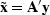A great many of the calculations we do in science and engineering are really matrix multiplication in disguise. The first goal of this chapter is to unmask the disguise by showing many examples. Second, we see how the adjoint operator (matrix transpose) back-projects information from data to the underlying model.

Geophysical modeling calculations generally use linear operators that predict data from models. Our usual task is to find the inverse of these calculations; i.e., to find models (or make maps) from the data. Logically, the adjoint is the first step and a part of all subsequent steps in this inversion process. Surprisingly, in practice the adjoint sometimes does a better job than the inverse! This is because the adjoint operator tolerates imperfections in the data and does not demand that the data provide full information.

Using the methods of this chapter, you will find that once you grasp the relationship between operators in general and their adjoints, you can obtain the adjoint just as soon as you have learned how to code the modeling operator.

If you will permit me a poet's license with words, I will offer you the following table of operators and their adjoints:


matrix multiply 		conjugate-transpose matrix multiply
convolve 		crosscorrelate
replicate, scatter, spray 		sum or stack
spray into neighborhood 		sum in bins
derivative (slope) 		negative derivative
causal integration 		anticausal integration
plane-wave superposition 		slant stack / beam form
superpose on a curve 		sum along a curve
stretch 		squeeze
upward continue 		downward continue
hyperbolic modeling 		normal moveout and CDP stack
diffraction modeling 		imaging by migration
ray tracing 		tomography


The left column above is often called modeling,'' and the adjoint operators on the right are often used in data processing.''

The adjoint operator is sometimes called the back projection'' operator because information propagated in one direction (earth to data) is projected backward (data to earth model). For complex-valued operators, the transpose goes together with a complex conjugate. In Fourier analysis, taking the complex conjugate ofreverses the sense of time. With more poetic license, I say that adjoint operators undo the time and phase shifts of modeling operators. The inverse operator does this too, but it also divides out the color. For example, when linear interpolation is done, then high frequencies are smoothed out, so inverse interpolation must restore them. You can imagine the possibilities for noise amplification. That is why adjoints are safer than inverses.

Later in this chapter we relate adjoint operators to inverse operators. Although inverse operators are more well known than adjoint operators, the inverse is built upon the adjoint so the adjoint is a logical place to start. Also, computing the inverse is a complicated process fraught with pitfalls whereas the computation of the adjoint is easy. It's a natural companion to the operator itself.

Much later in this chapter is a formal definition of adjoint operator. Throughout the chapter we handle an adjoint operator as a matrix transpose, but we hardly ever write down any matrices or their transposes. Instead, we always prepare two subroutines, one that performsand another that performs.So we need a test that the two subroutines really embody the essential aspects of matrix transposition. Although the test is an elegant and useful test and is itself a fundamental definition, curiously, that definition does not help construct adjoint operators, so we postpone a formal definition of adjoint until after we have seen many examples.